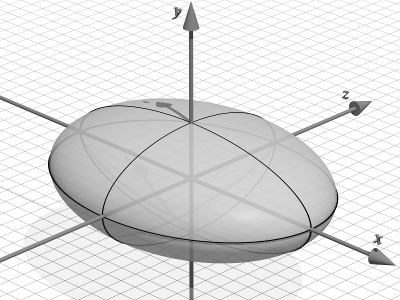# Ellipsoid

(diff) ← Older revision | Latest revision (diff) | Newer revision → (diff)

(real)

2010 Mathematics Subject Classification: Primary: 51-XX [MSN][ZBL]Figure 1. An ellipsoid with $a$, $b$, $c$ in the ratio 8:3:6 (pdf)

An ellipsoid is a closed central surface of the second order (see Figure 1).

The canonical equation of an ellipsoid has the form $$\frac{x^2}{a^2} + \frac{y^2}{b^2} + \frac{z^2}{c^2} = 1$$ The positive numbers $a$, $b$ and $c$ and the segments of corresponding lengths are called the semi-axes of the ellipsoid. The section of an ellipsoid by any plane is an ellipse.

If two semi-axes of an ellipsoid are equal, the ellipsoid is called an ellipsoid of revolution, and the sections of an ellipsoid of revolution by planes parallel to the plane of the equal semi-axes are circles. When $a=b=c$, the ellipsoid is a sphere. The centre of symmetry of an ellipsoid is called the centre of the ellipsoid.

A second-order surface with canonical equation $$\frac{x^2}{a^2} + \frac{y^2}{b^2} + \frac{z^2}{c^2} = 1$$ is called an imaginary ellipsoid.